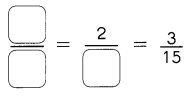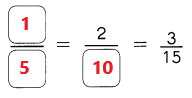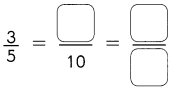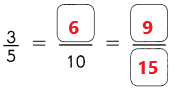# Math in Focus Grade 3 Chapter 14 Practice 2 Answer Key Understanding Equivalent Fractions

Practice the problems of Math in Focus Grade 3 Workbook Answer Key Chapter 14 Practice 2 Understanding Equivalent Fractions to score better marks in the exam.

## Math in Focus Grade 3 Chapter 14 Practice 2 Answer Key Understanding Equivalent Fractions

Example

Shade the part(s) to show fractions equivalent to $$\frac{1}{4}$$. Then write the fractions.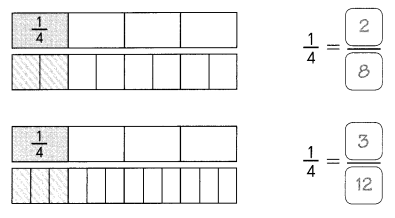Question 1.
Shade the part(s) to show fractions equivalent to $$\frac{1}{5}$$. Then write the fractions.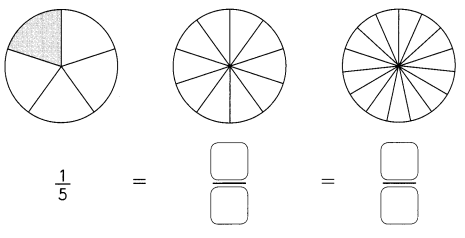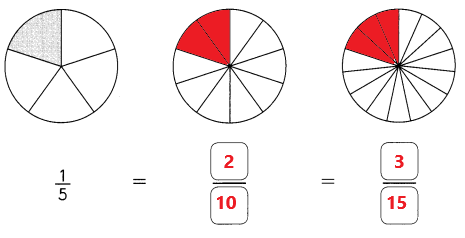Explanation:
In the above diagram we can observe 3 images.
In first image we can observe one – fifth part is shaded.
The given fraction is 1/5. Multiply numerator and denominator with 2. The equivalent fraction for 1/5 is 2/10. So, 2/10 part is shaded in second image.
The given fraction is 1/5. Multiply numerator and denominator with 3. The equivalent fraction for 1/5 is 3/15. So, 3/15 part is shaded in third image.

Question 2.
Divide the second bar into 10 equal parts. Shade the part(s) to show a fraction equivalent to $$\frac{2}{5}$$. Write the fraction.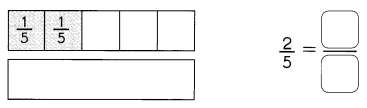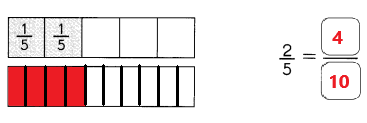Explanation:
In the above image we can observe two bars.
The first bar is divided into 5 equal parts. In the first bar 2/5 part is shaded.
We have to divide second bar into 10 equal parts. The equivalent fraction for 2/5 is 4/10. In second bar 4/10 part is shaded with red color.

Question 3.
Divide the second bar into 12 equal parts. Shade the part(s) to show a fraction equivalent to $$\frac{5}{6}$$. Write the fraction.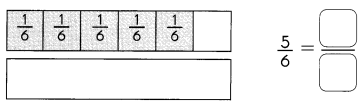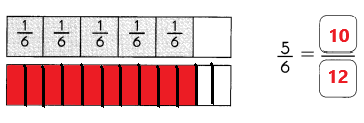Explanation:
In the above image we can observe two bars.
The first bar is divided into 6 equal parts. In the first bar 5/6 part is shaded.
We have to divide second bar into 12 equal parts. The equivalent fraction for 5/6 is 10/12. In second bar 10/12 part is shaded with red color.

Question 4.
Find the missing numerator or denominator.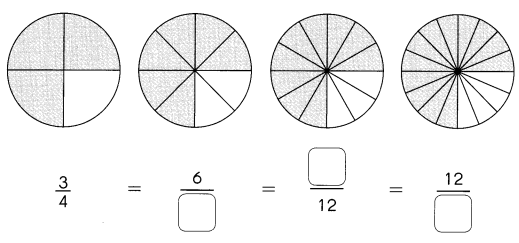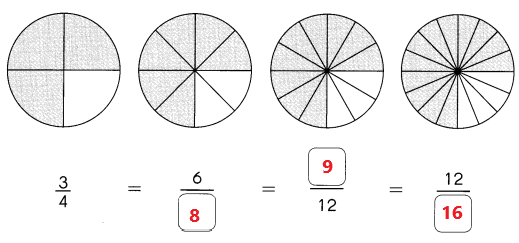Explanation:
In the above diagram we can observe four images.
In first image 3/4 part is shaded.
In second image 6/8 part is shaded. The missing denominator is 8.
In third image 9/12 part is shaded. The missing numerator is 9.
In fourth image 12/16 part is shaded. The missing denominator is 16.

Use number lines to find equivalent fractions.

Example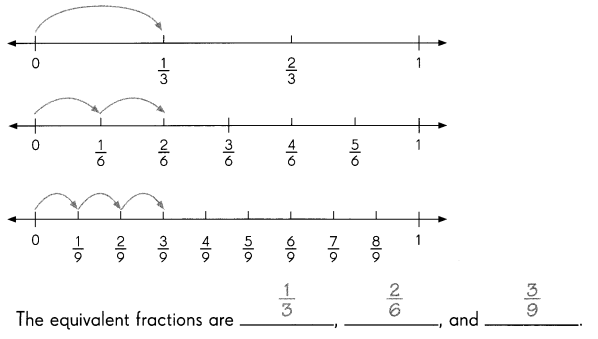Question 5.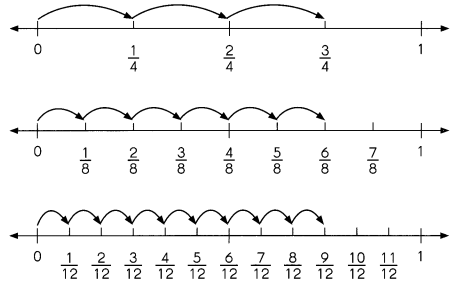The equivalent fractions are __________, _________, and __________.
The equivalent fractions are 3/4, 6/8, and 9/12.
Explanation:
In the above image we can observe three number lines.
In first number line we can observe 3/4. In the second number line we can observe 6/8. In third number line we can observe 9/12. The equivalent fractions are 3/4, 6/8, and 9/12.
Fill in the missing tractions on the number lines Then use the number lines to find the missing numerators and denominators.

Question 6.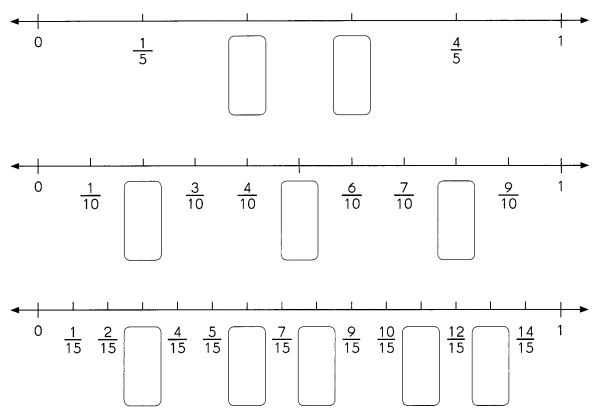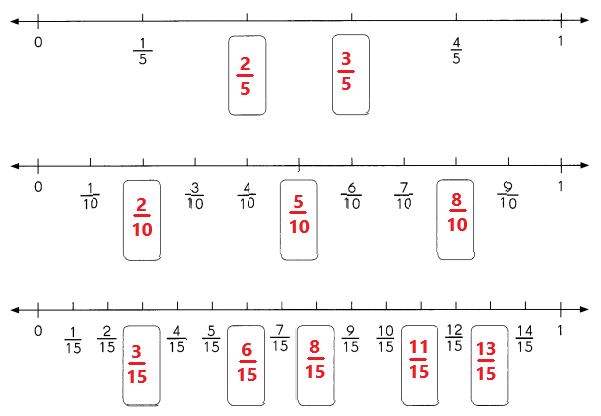Explanation:
In the above image we can observe three number lines.
In first number line we can observe some fractions are missing. The missing fractions are 2/5 and 3/5.
In second number line also we can observe some fractions are missing. The missing fractions are 2/10, 5/10 and 8/10.
In third number line also we can observe some fractions are missing. The missing fractions are 3/15, 6/15, 8/15, 11/15 and 13/15.

Question 7.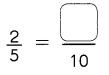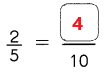Explanation:
In first number line we can observe 2/5. In second number line we can observe 4/10 exactly below to the fraction 2/5. The equivalent fraction for 2/5 is 4/10.The missing numerator is 4.

Question 8.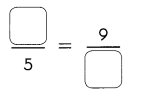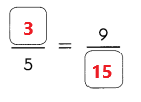Explanation:
In first number line we can observe 3/5. In third number line we can observe 9/15 exactly below to the fraction 3/5. The equivalent fraction for 3/5 is 9/15.The missing numerator is 3. The missing denominator is 15.

Question 9.